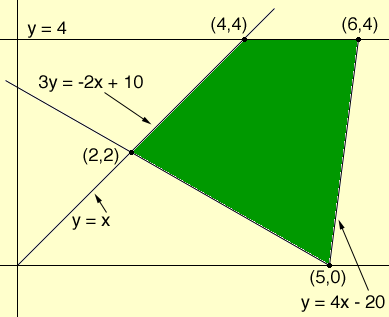SEARCH HOMEMath Central Quandaries & QueriesQuestion from Sylvia, a student: What is graphing linear programming?Hi Sylvia,

In a linear programming problem you are to find the maximum or minimum of a linear function, called the objective function, where there are constraints which are also given as linear functions. If there are two independent variables involved then the constraint equations are lines in the plane and the problem can be solved graphically. Let me illustrate with an example.

Suppose you are to find the maximum of the function

z = 5x + 3y - 6

subject to the constraints

y ≤ x
y≤ 4
3y ≥ -2x + 10
y ≥ 4x - 20

The region of the plane described by these inequalities, called the feasible region isThe main theorem of linear programming states that any maximum value of the objective function occurs at one of the vertices of the feasible region. Thus to find the maximum value of

z = 5x + 3y - 6

subject to the constraints given you only need to evaluate this function at (2,2), (5,0), (6,4) and (4,4) and select the largest value.

PennyMath Central is supported by the University of Regina and The Pacific Institute for the Mathematical Sciences.